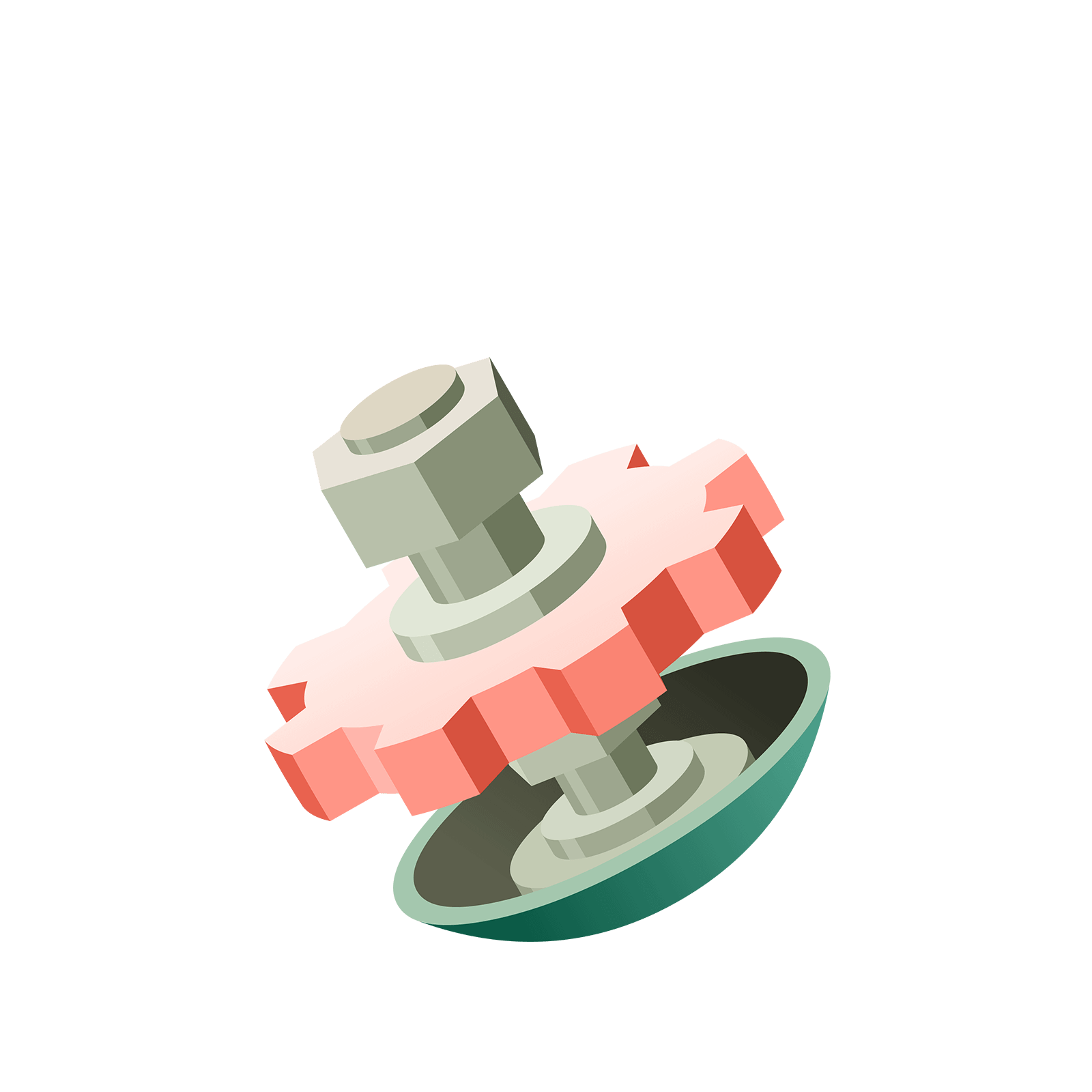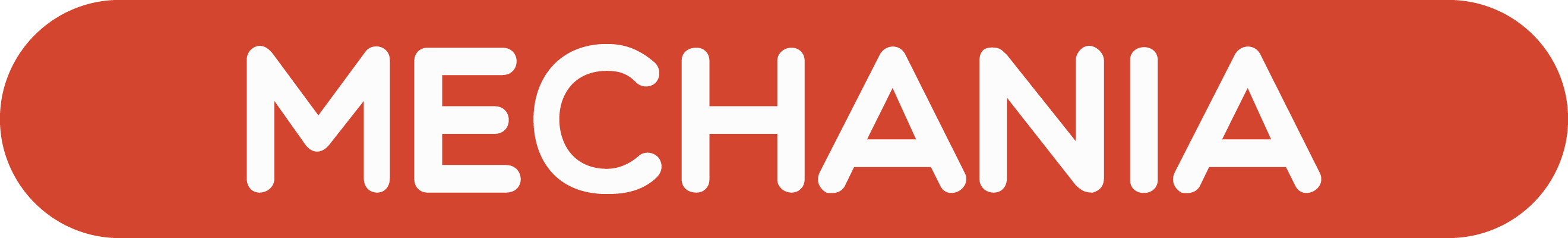06 026 368# Station  AMore MachinesAnd why stop at a $1 \leftarrow 3$ machine?

### Question 1

What do you think the rule is for a $1 \leftarrow 4$ machine?
Can you predict what the $1 \leftarrow 4$ machine code for thirteen shall be?### Question 2

What is the $1 \leftarrow 5$ code for number thirteen?### Question 3

What is the $1 \leftarrow 9$ code for number thirteen?### Question 4

What is the $1\leftarrow 5$ code for number twelve?### Question 5

What is the $1\leftarrow 9$ code for the number twenty?Is it weird that two different numbers have the same code in two different machines?